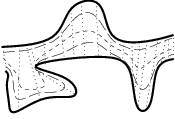## Morphing between Polylines

#### Alon Efrat, Leonidas J. Guibas, Sariel Har-Peled, and T. M. Murali.

Abstract:

Given two non-intersecting simple polylines in the plane, we study the problem of continuously transforming or morphing one polyline into the other. Our morphing strategies have the desirable property that every intermediate polyline is also simple. We also guarantee that no portion of the polylines to be morphed is stretched or compressed by more than a user-defined parameter during the entire morphing. Our algorithms are based on the morphing width, a new metric we have developed for measuring the similarity between two polylines. We develop an algorithm that computes the morphing width of the two polylines and constructs a corresponding morphing strategy in $O\left(n2log2n\right)$ time using $O\left(n2\right)$ space, where $n$ is the total number of vertices in the polylines. We describe another algorithm that computes a factor-$2$ approximation of the morphing width and a corresponding morphing scheme in $O\left(n log n\right)$ time.